# How to Split Data in Long Column into Multiple Columns in Excel

Sometimes we need to split data from a long column into a table with several columns based on some criteria like X rows or X columns. If we split data by manual, it is very complex and troublesome. So, we need a simple way to split data into multiple columns. This article will introduce you two convenient ways to split data, one is by VBA script. And then after running macro, you can get a dialog to type your demands, then it can help you to split data automatically and correctly. The second way is by formula. This way is quite helpful for those users who are not skilled in coding. You can select one you like. Now let’s get started.

Prepare a long list in one column in excel. See example below. This is a list of names.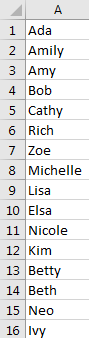If we want to split them into 4 rows, we can follow below steps.

## Method 1: Split Data into Multiple Columns by VBA in Excel

Step 1: On current visible worksheet, right click on sheet name tab to load Sheet management menu. Select View Code, Microsoft Visual Basic for Applications window pops up.

Or you can enter Microsoft Visual Basic for Applications window via Developer->Visual Basic.

Step 2: In Microsoft Visual Basic for Applications window, click Insert->Module, enter below code in Module1:

```Sub SplitDataintoMultipleColumns()

Dim rng As Range

Dim InputRng As Range

Dim OutputRng As Range

Dim xRow As Integer

Dim xCol As Integer

Dim xArr As Variant

Set InputRng = Application.Selection

Set InputRng = Application.InputBox("Select Input Range :", "SplitDataintoMultipleColumns", InputRng.Address, Type:=8)

xRow = Application.InputBox("Enter Row Number :", "SplitDataintoMultipleColumns")

Set OutputRng = Application.InputBox("Select Output Range :", xTitleId, Type:=8)

Set InputRng = InputRng.Columns(1)

xCol = InputRng.Cells.Count / xRow

ReDim xArr(1 To xRow, 1 To xCol + 1)

For i = 0 To InputRng.Cells.Count - 1

xValue = InputRng.Cells(i + 1)

iRow = i Mod xRow

iCol = VBA.Int(i / xRow)

xArr(iRow + 1, iCol + 1) = xValue

Next

OutputRng.Resize(UBound(xArr, 1), UBound(xArr, 2)).Value = xArr

End Sub```

Step 3: Save the codes, see screenshot below. And then quit Microsoft Visual Basic for Applications.

Step 4: Click Developer->Macros to run Macro. Select ‘SplitDataintoMultipleColumns’ and click Run.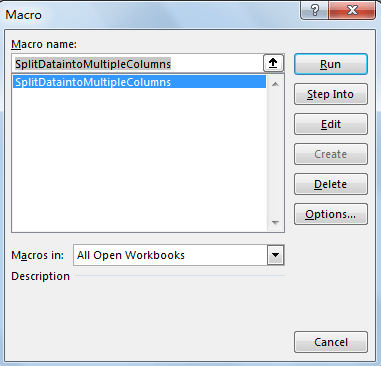Step 5: Split Data dialog pops up. Enter Select Input Range \$A\$1:\$A\$16. Click OK. In this step you can select the long list range you want to do split.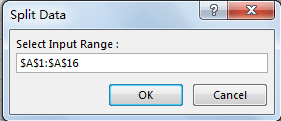Step 6: Enter 4 into “Enter Row Number”. Click OK. In this step you can enter the row number per your demands.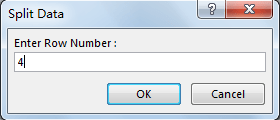Step 7: On Split Data dialog, Enter Select Output Range \$D\$1. Click OK. Please be aware that you can select only one single cell is ok. In this case, we select D1 as output range, then split data will be listed in column D started from D1, as we entered row number as 4 in last step, so only D1 to D4 will save the split data, then left data will be listed in E column into 4 rows.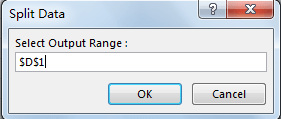Step 8: Click OK. Verify that data is split to 4 rows properly.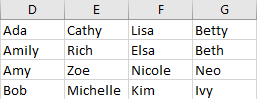Step 9: You can also change row number to other value, table will be created per your input automatically. For example, enter row number as 9, you will get below table.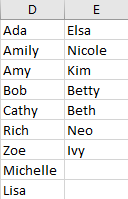## Method 2: Split Data into Multiple Columns by Formula in Excel

This method is friendly to users who are not good at coding. Just copy the formula and use it.

Step 1: In C1 enter the formula =INDEX(\$A:\$A,ROWS(\$A\$1:A1)+(COLUMNS(\$A\$1:A1)-1)*4). In this formula, 4 in (COLUMNS(\$A\$1:A1)-1)*4) is the row number you want to split data into the new range. You can change to other value you like.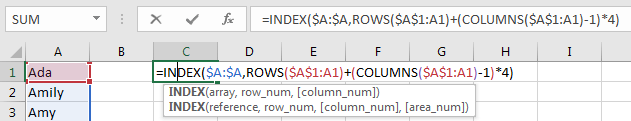Step 2: Click Enter. Verify that value in A1 is listed in C1.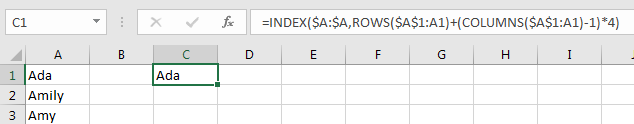Step 3: Drag the fill handle to row 4 and then across to other columns like D, E, F. Verify that data is split to 4 rows properly.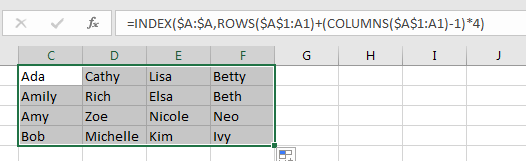Step 4: Change 4 rows to 5 rows and check again. Verify that data is split to 5 rows properly, for the cells without value, 0 displays.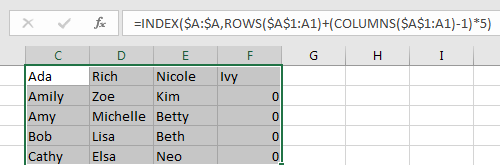Related Posts

Find and Replace Multiple Values

This post will guide you how to find and replace multiple values at once with VBA macro or using formula  in Excel. How do I make multiple find and replace in Excel. Suppose that you have a few cells containing ...

VBA Macro For VLOOKUP From Another Sheet

In the previous post, you should know that how to fix or remove the #N/A error when using VLOOKUP formula to lookup value from another sheet. And this post will show you how to use VBA code to vlookup data ...

How To Insert Comments in Protected Worksheet in Excel

This post will show you how to allow comments in a protected worksheet in Excel. You can easily to insert comments into cells in a normal worksheet in Excel, but if want to insert a comment in a worksheet that ...

How To Convert Text to Upper Cases(Using VBA) in Excel

This post will show you how to switch from lower case to upper case in Excel. and I am going to show you two different ways of converting text to upper cases using formula or VBA macro in Excel 2013,Excel ...

How To Hide Every Other Row in Excel (Using VBA)

This post will show you how to hide alternate rows or columns in Excel or how to hide every third, fourth, fifth row or column in Excel. If you want to hide every other row in your current worksheet, how ...

How to Disable the Save As Prompt in Excel

This post will show you how to use a VBA Macro to save an Excel file and overwrite any existing file without a prompt so that you are going to get the little window that says file already exists do ...

How to Count Cells that Contain even or odd numbers in Excel

This post will guide you how to count the number of cells that contain odd or even numbers within a range of cells using a formula in Excel 2013/2016.How do I count cells that contain odd numbers through the use ...

How to Count Cells that Contain negative Numbers in Excel

This post will guide you how to count the number of cells that contain negative numbers within a range of cells using a formula in Excel 2013/2016.You can count the number of negative numbers in your data using easy functions ...

How to Count Cells Are Not Blank or Empty in Excel

This post will guide you how to count cells that are not blank or empty in a given range cells using a formula in Excel 2013/2016.How do I count the number of cells that are not blank in a particular ...

How to Count Cells Less Than a Specific Value in Excel

This post will guide you how to count the number of cells less than a particular numeric value in a given range cells using a formula in Excel 2013/2016. How do I count cells that are less than a specific ...

Sidebar